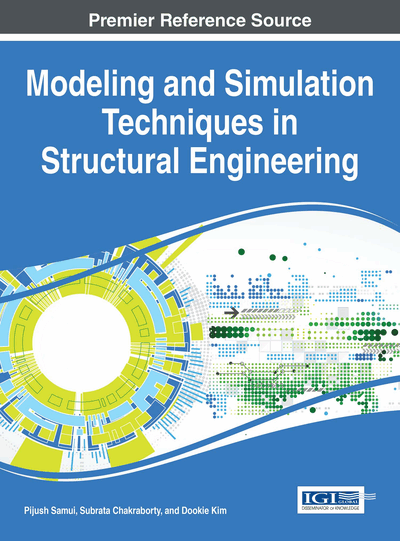# Fuzzy Structural Analysis Using Surrogate Models

A. S. Balu (National Institute of Technology Karnataka, India) and B. N. Rao (Indian Institute of Technology Madras, India)
DOI: 10.4018/978-1-5225-0588-4.ch008

## Abstract

The exponential growth of computational power during the last few decades has enabled the finite element analysis of many real-life engineering systems which are too complex to be analytically solved in a closed form. In the traditional deterministic finite element analysis, system parameters such as mass, geometry and material properties are assumed to be known precisely and defined exactly. However, in practice most of the data used in the solution process of many practical engineering systems are either collected from experiments or acquired as empirical data from the past, which are usually ill defined, imprecise and uncertain in nature. This work presents a practical approach based on High Dimensional Model Representation (HDMR) for analyzing the response of structures with fuzzy parameters. The proposed methodology involves integrated finite element modelling, HDMR based surrogate model, and explicit fuzzy analysis procedures.
Chapter Preview
Top

## Introduction

The uncertainties present in the system can be categorized into randomness and fuzziness. Probabilistic methods are especially suitable in case of uncertainty due to randomness for which the information on both the range and the probability density function are available. The probabilistic concept is already well established for the extension of the deterministic finite element method towards uncertainty assessment. This has led to a number of probabilistic finite element procedures (Haldar & Mahadevan, 2000; Lernout et. al., 2006; Pellissetti & Schueller, 2007; Schueller et. al., 2004; Soize, 2009) that are often used in conjunction with Monte Carlo simulations. However, the use of a probabilistic approach can lead to subjective results if the amount of statistical data on the uncertainties is limited, and fuzzy information that is vague, imprecise, qualitative, linguistic or incomplete need to be included (Elishakoff, 1995). The need to incorporate subjective information into the design and analysis of engineering systems has led to the development of fuzzy finite element methodology that began in the early 1990s.

Fuzzy set theory initiated by Zadeh (1965) provides a concept for the description of linguistic or subjective knowledge and incomplete data in a non-probabilistic manner. The use of Zadeh’s concept in the finite element context has led to the development of the fuzzy finite element method for the solution of engineering problems (Dhingra et. al., 1992; Valliappan & Pham, 1995; Chen & Rao, 1997; Akpan et.al., 2001a; Akpan et.al., 2001b). Its aim is to obtain the membership function of an output quantity, based on the fuzzy description of the uncertain input parameters. By using the-level technique, the interval finite element method forms the core of the fuzzy procedure (Gersem et. al., 2007). Over the last decade, several interval FE procedures have been reported, such as the vertex method (Dong & Shah, 1987; Hanss, 2002; Donders et. al., 2005; Gauger et al., 2008), the global optimization approaches (Valliappan & Pham, 1995; Möller et. al., 2000; Farkas et. al., 2008) and the interval arithmetic approaches (Rao & Berke, 1997; Qiu & Elishakoff, 1998; Dessombz et. al., 2001; Muhanna & Mullen, 2001). However, each of these techniques has its specific limitations when applied to realistic engineering problems, and none of them has been established as standard interval procedure, and the choice of technique depends on the type of the conducted FE analysis.

This work presents a practical approach based on High Dimensional Model Representation (HDMR) (Li et. al., 2001a; Li et. al., 2001b; Sobol, 2003; Chowdhury et. al., 2008; Balu & Rao, 2012a; Balu & Rao, 2012b; Balu & Rao, 2012c) for analyzing the response of structures with fuzzy parameters. The proposed methodology involves integrated finite element modelling, HDMR based response surface generation, and explicit fuzzy analysis procedure. The uncertainties in the material, geometric, loading and structural parameters are represented using fuzzy sets. To facilitate efficient computation, a HDMR based response surface generation is employed for the approximation of the fuzzy finite element response quantity, and the response is expressed in a separable closed-form expression as a linear combination of the fuzzy variables through the definition of intervening variables, then using the transformation technique the bounds on the response at each α-level are obtained.

## Complete Chapter List

Search this Book:
Reset